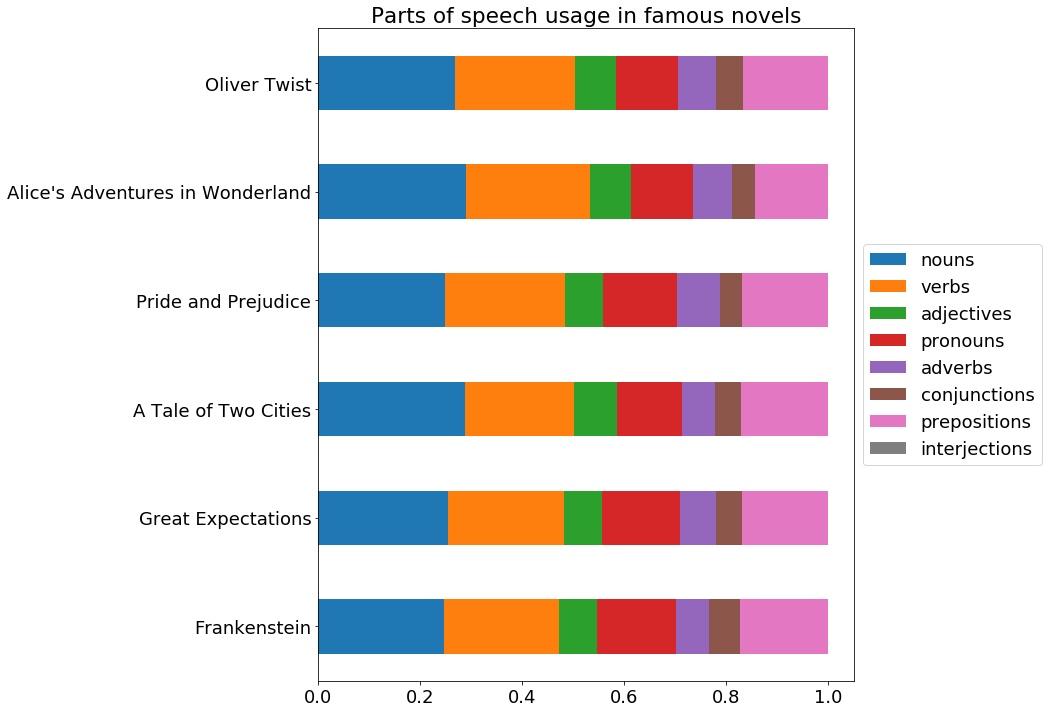This notebook takes off from Visualize Parts of Speech 1, which ended with a visualization from a single text. In this notebook, we look at how to visually compare the part of speech usage in many texts.

from urllib import request
import nltk
import os
import pandas as pd
import matplotlib
import matplotlib.pyplot as plt
import re
import get_gutenberg


We’re going to use the class for gathering text we made previously.

books = [get_gutenberg.Book('Frankenstein', 'http://www.gutenberg.org/cache/epub/84/pg84.txt', 500),
get_gutenberg.Book('Great Expectations', 'http://www.gutenberg.org/files/1400/1400-0.txt', 885),
get_gutenberg.Book('A Tale of Two Cities', 'https://www.gutenberg.org/files/98/98-0.txt', 2400),
get_gutenberg.Book('Pride and Prejudice', 'https://www.gutenberg.org/files/1342/1342-0.txt', 1200),
get_gutenberg.Book("Alice's Adventures in Wonderland", 'https://www.gutenberg.org/files/11/11-0.txt', 1200),
get_gutenberg.Book('Oliver Twist', 'http://www.gutenberg.org/cache/epub/730/pg730.txt', 500)]

# We'll grab this function from the Part of Speech notebook
def pos_plotter(parts_of_speech):
'''This function inputs a specific list of lists that is shown in the Part of Speech notebook'''
all_labels = ['common_nouns', 'verbs', 'adjectives', 'pronouns', 'adverbs', 'proper_nouns', 'conjunctions', 'prepositions', 'interjections', 'modals']
pos_dict = dict(zip(all_labels, parts_of_speech))
labels = []
data = []
for pos, lst in pos_dict.items():
if lst:
data.append(len(lst))
labels.append(pos)
fig1, ax1 = plt.subplots()
ax1.pie(data, labels=labels)
ax1.axis('equal')  # Equal aspect ratio ensures that pie is drawn as a circle.
plt.show()

titles = []
for book in books:
titles.append(book.title)
print(titles)

['Frankenstein', 'Great Expectations', 'A Tale of Two Cities', 'Pride and Prejudice', "Alice's Adventures in Wonderland", 'Oliver Twist']

for book in books:
pos_plotter(book.pos)

Frankenstein file already exists
Extracting text from file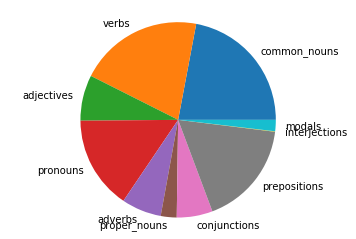Great Expectations file already exists
Extracting text from file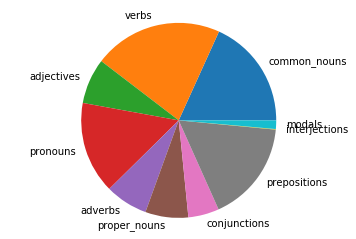A Tale of Two Cities file already exists
Extracting text from file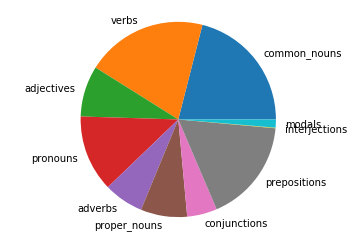Pride and Prejudice file already exists
Extracting text from file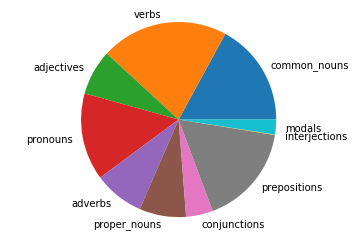Alice's Adventures in Wonderland file already exists
Extracting text from file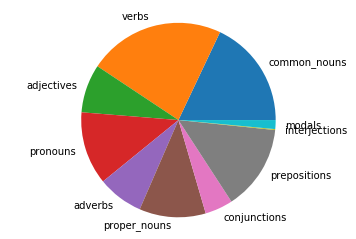Oliver Twist file already exists
Extracting text from file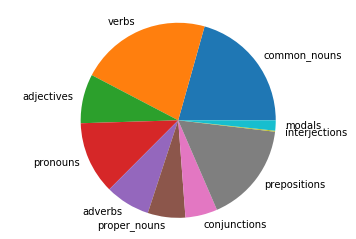It is not easy to compare the relative part of speech usage using pie charts. A better way would be to make a horizontal bar chart for each book and then stack them vertically to compare. Let’s try that.

# We'll grab this function from the Part of Speech notebook
import matplotlib.pyplot as plt
def pos_plotter2(parts_of_speech):
'''This function inputs a specific list of lists that is shown in the Part of Speech notebook'''
all_labels = ['common_nouns', 'verbs', 'adjectives', 'pronouns', 'adverbs', 'proper_nouns', 'conjunctions', 'prepositions', 'interjections', 'modals']
pos_dict = dict(zip(all_labels, parts_of_speech))
labels=[]
data=[]
for pos, lst in pos_dict.items():
if lst:
data.append(len(lst))
labels.append(pos)
fig1, ax1 = plt.subplots()
ax1.bar(data, labels=labels)
ax1.axis('equal')  # Equal aspect ratio ensures that pie is drawn as a circle.
plt.show()

nouns = []
verbs = []
pronouns = []
conjunctions = []
prepositions = []
interjections = []
modals = []
for book in books:
nouns.append(len(book.pos) + len(book.pos)) # common + proper
verbs.append(len(book.pos) + len(book.pos)) # verbs + modals
pronouns.append(len(book.pos))
conjunctions.append(len(book.pos))
prepositions.append(len(book.pos))
interjections.append(len(book.pos))

# Now let's put everything in a pandas dataframe
'conjunctions': conjunctions, 'prepositions': prepositions, 'interjections': interjections})

df_norm = df.div(df.sum(axis=1), axis='index')

0 0.246683 0.224138 0.075597 0.154036 0.065970 0.059315 0.173450 0.000812
1 0.253488 0.228029 0.075317 0.152568 0.070661 0.051144 0.167822 0.000970
2 0.287067 0.214025 0.084292 0.126739 0.065866 0.049669 0.171285 0.001057
3 0.248096 0.235704 0.075024 0.144551 0.083393 0.044378 0.167871 0.000983
4 0.290086 0.241904 0.080600 0.121415 0.076145 0.046469 0.141839 0.001542

We’ll need a function that will help convert the axes to percentages. Let’s make that now.

fig, ax = plt.subplots()
fig.set_size_inches(12, 12)
matplotlib.rcParams.update({'font.size': 18})

df_norm.plot.barh(stacked=True, ax=ax)

ax.set_title("Parts of speech usage in famous novels")

# Shrink current axis by 20%
box = ax.get_position()
ax.set_position([box.x0, box.y0, box.width * 0.8, box.height])